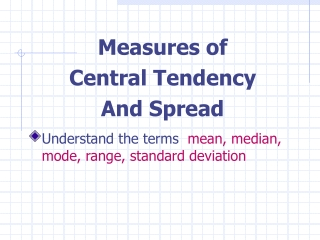DownloadDownload PresentationMeasures of Central Tendency And Spread

# Measures of Central Tendency And Spread

Télécharger la présentation## Measures of Central Tendency And Spread

- - - - - - - - - - - - - - - - - - - - - - - - - - - E N D - - - - - - - - - - - - - - - - - - - - - - - - - - -
##### Presentation Transcript

1. Measures of Central Tendency And Spread • Understand the terms mean, median, mode, range, standard deviation

2. Measures of Central Tendency • Mean … the average score • Median … the value that lies in the middle after ranking all the scores • Mode … the most frequently occurring score

3. Measures of Central Tendency Which measure of Central Tendency should be used?

4. Measures of Central Tendency The measure you choose should give you a good indication of the typical score in the sample or population.

5. Measures of Central Tendency Mean … the most frequently used but is sensitive to extreme scores e.g. 1 2 3 4 5 6 7 8 9 10 Mean = 5.5 (median = 5.5) e.g. 1 2 3 4 5 6 7 8 9 20 Mean = 6.5 (median = 5.5) e.g. 1 2 3 4 5 6 7 8 9 100 Mean = 14.5 (median = 5.5)

6. Measures of Central Tendency Median … is not sensitive to extreme scores … use it when you are unable to use the mean because of extreme scores

7. Measures of Central Tendency Mode … does not involve any calculation or ordering of data … use it when you have categories (e.g. occupation)

8. Variation or Spread Measures that indicate the spread of scores: • Range • Standard Deviation

9. Variation or Spread of Distributions Range • It compares the minimum score with the maximum score • Max score – Min score = Range • It is a crude indication of the spread of the scores because it does not tell us much about the shape of the distribution and how much the scores vary from the mean

10. Variation or Spread of Distributions Standard Deviation • It tells us what is happening between the minimum and maximum scores • It tells us how much the scores in the data set vary around the mean • It is useful when we need to compare groups using the same scale

11. Calculating a Mean and a Standard Deviation